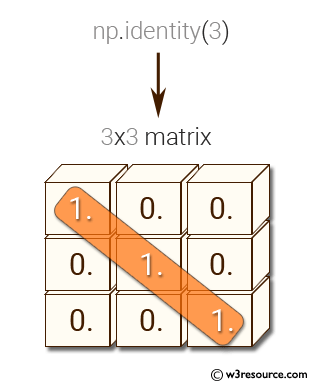﻿ NumPy: Create a 3x3 identity matrix - w3resource

# NumPy: Create a 3x3 identity matrix

## NumPy: Basic Exercise-16 with Solution

Write a NumPy program to create a 3x3 identity matrix.

Sample Solution :

Python Code :

``````import numpy as np
array_2D=np.identity(3)
print('3x3 matrix:')
print(array_2D)
``````

Sample Output:

```3x3 matrix:
[[ 1.  0.  0.]
[ 0.  1.  0.]
[ 0.  0.  1.]]
```

Pictorial Presentation:Python Code Editor:

Have another way to solve this solution? Contribute your code (and comments) through Disqus.

What is the difficulty level of this exercise?

﻿

Inviting useful, relevant, well-written and unique guest posts